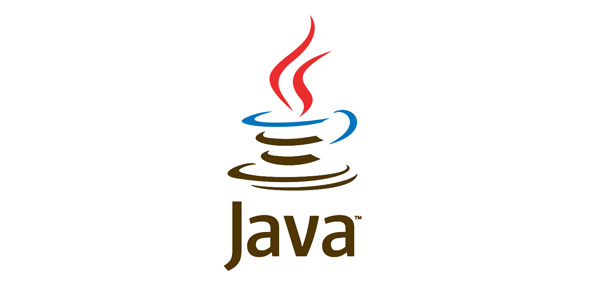# Java Ch 2 Quiz

11 Questions | Total Attempts: 537SettingsCreate your own Quiz• 1.
The ____ rules of a language determine which instructions are valid.
• 2.
True or False: Hello! is an example of a legal identifier.
• A.

True

• B.

False

• 3.
True or False: The Boolean data type has two possible values – true and false.
• A.

True

• B.

False

• 4.
What is the value of the following expression?
• 5.
If an operator has an integer and a floating-point operand, the result of the operation is a ____ number.
• 6.
The expression (int)9.2 evaluates to ____.
• 7.
True or False: The value of a variable can change during execution.
• A.

True

• B.

False

• 8.
Suppose a and b are int variables. What is the value of b? a = 3; b = 4 + (++a)
• 9.
Java always initializes the value of a variable.
• A.

True

• B.

False

• 10.
Given the following assignments. What is the value in str? String str; str = “Hello”; str = str + “ World”;
• 11.
A ____ is a set of instructions designed to accomplish a specific task.
Related TopicsBack to top
×

Wait!
Here's an interesting quiz for you.